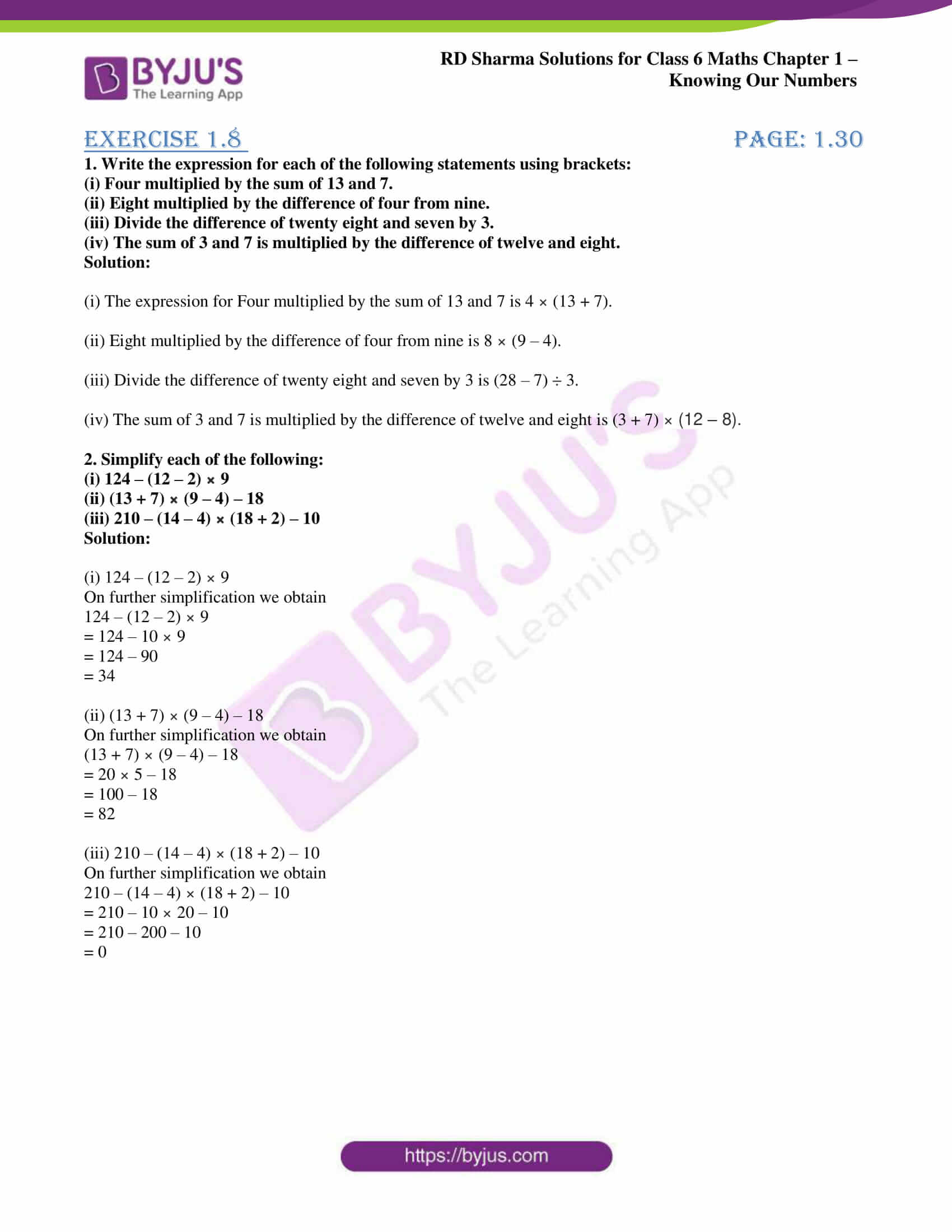# RD Sharma Solutions for Class 6 Maths Chapter 1: Knowing Our Numbers Exercise 1.8

## RD Sharma Solutions for Class 6 Maths Exercise 1.8 PDF

The solutions PDF which are created by the teachers at BYJU’S help students improve their knowledge about the concepts covered in the Chapter. The primary objective of providing students with exercise wise solutions is to help them with solving problems accurately. They provide students with a thorough understanding of concepts based on the marks weightage in CBSE syllabus. The solutions are designed by subject experts after conducting research on each topic. The students can easily download PDF and use them as a reference guide to perform well in the exam. RD Sharma Solutions for Class 6 Maths Chapter 1 Knowing Our Numbers Exercise 1.8 are provided here.

## RD Sharma Solutions for Class 6 Maths Chapter 1: Knowing Our Numbers Exercise 1.8 Download PDF## Access answers to Maths RD Sharma Solutions for Class 6 Chapter 1: Knowing Our Numbers Exercise 1.8

#### Exercise 1.8 page: 1.30

1. Write the expression for each of the following statements using brackets:

(i) Four multiplied by the sum of 13 and 7.

(ii) Eight multiplied by the difference of four from nine.

(iii) Divide the difference of twenty eight and seven by 3.

(iv) The sum of 3 and 7 is multiplied by the difference of twelve and eight.

Solution:

(i) The expression for Four multiplied by the sum of 13 and 7 is 4 × (13 + 7).

(ii) Eight multiplied by the difference of four from nine is 8 × (9 – 4).

(iii) Divide the difference of twenty eight and seven by 3 is (28 – 7) ÷ 3.

(iv) The sum of 3 and 7 is multiplied by the difference of twelve and eight is (3 + 7) × (12 – 8).

2. Simplify each of the following:

(i) 124 – (12 – 2) × 9

(ii) (13 + 7) × (9 – 4) – 18

(iii) 210 – (14 – 4) × (18 + 2) – 10

Solution:

(i) 124 – (12 – 2) × 9

On further simplification we obtain

124 – (12 – 2) × 9

= 124 – 10 × 9

= 124 – 90

= 34

(ii) (13 + 7) × (9 – 4) – 18

On further simplification we obtain

(13 + 7) × (9 – 4) – 18

= 20 × 5 – 18

= 100 – 18

= 82

(iii) 210 – (14 – 4) × (18 + 2) – 10

On further simplification we obtain

210 – (14 – 4) × (18 + 2) – 10

= 210 – 10 × 20 – 10

= 210 – 200 – 10

= 0

### RD Sharma Solutions Class 6 Maths Chapter 1 – Knowing Our Numbers Exercise 1.8

RD Sharma Solutions Class 6 Maths Chapter 1 Knowing Our Numbers Exercise 1.8 explains the concept of using brackets while addition, subtraction, multiplication and division in exercise wise problems.

### Key features of RD Sharma Solutions for Class 6 Maths Chapter 1: Knowing Our Numbers Exercise 1.8

• RD Sharma Solutions help students obtain a clear idea of concepts which are covered in each exercise.
• It is very helpful for learning the Chapter thoroughly and for revision purpose from the exam point of view.
• The students get familiar with the types of questions which can be asked in the exam and the methods of solving them.
• Solving the exercise wise problems help students in answering the questions with more accuracy based on CBSE syllabus.